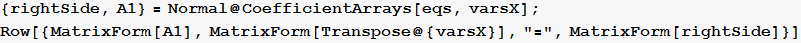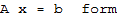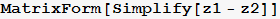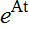Symbolic solution to expansion of natural frequencies method for determiningWritten by Nasser M. Abbasi, based on lecture given by Professor Barmish, ECE 717, University of Wisconsin, Madison
11/1/2014

The method of findingusing expansion of natural frequencies is first applied to a 2×2 matrix to illustrate the symbolic method. Then a general purpose function is written at the end using the symbolic method and applied to much larger problems for illustrations. This is a standalone function which can be called to determinefor matrices A which can have repeated eigenvalues.  The result is verified by comparing the output to Mathematica buildin function MatrixExp[]

Example 1 from lecture done using symbolics

Define AFind eigenvalues of ASet the eigenvalue multiplersApplyalgorithm using expansion of natural frequenciesAutomatic generation of  lists of unknowns Y(k,i) and list of symbols to b[i] to use for solving Ax=bTake repeated derivatives ofto generate the set of equations to solve for Y(k,i)The problem can be viewed as Ax=b where x=Y(k,i) is the unknowns and b are the symbolic names for the matrix powers.Now solve Ax=bReplace symbols b(i) on the right of the above, by the actual numerical values of the matrix powersNow apply these numerical values to the solution found earlier in order to obtain numerical values for Y(k,i)Now since Y(k,i) are now found,  evaluatefound above using the above solutionCompare to Mathematica buildin functionFunction to automatically calculateusing symbolic natural frequencies methodExample 2 from lecture solved using the above functionλ multiplier 3 1 2 3 1 2Compare to buildin function to verifyVerification Method, suggested by Professor Barmish. We Differentiate Matrixfound above and see it is the same asfor t=0k012345